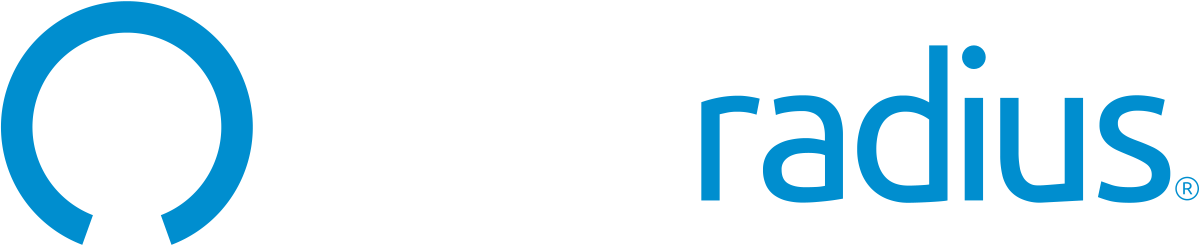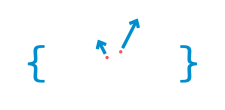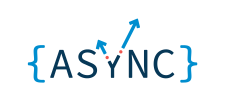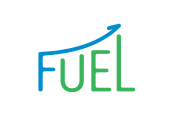Join us on the demo, while our product experts provide a detailed walkthrough of our enterprise platform.The latest news in the world of engineering.Identity and Access Management (IAM), including security and customer experience.

Visit BlogVisit Blog

# Full data science pipeline implementation

Learn how to implement the full data science pipeline right from collecting the data to implementing ML algorithms.Rinki Nag
October 09, 2020

## What is data enrichment? and its importance

Data enrichment is the process of combining first-party data from internal sources with disparate data from other internal systems or third-party data from external sources.

Usually, the data available from clients or stakeholders are not enough to solve the given problem statement, like if a client comes with a problem statement to build a recommendation engine for his mutual fund industry, the usual data they have is old purchase data but that's not enough as client behaviour changes with time and is impacted by the present market condition, oil prices, etc. which needs to be incorporated in the model to make it efficient.

Codes for this tutorial is at Link

The whole process id divided into four steps:

I have implemented a full pipeline of data science from scrapping data from web to implementing ml and NLP classification.

• Phase I:

Here I have scraped data from IMDB website (imdb.py)

• Phase II:

I have tried to implement simple ML regression on the data (ml_imdb.py)

• Phase III:

I have prepared the data for NLP classification (multilabel_prep.py)

• Phase IV:

I have implemented multilabel NLP classifier using various techniques like chain classifier etc. (multilabelnlpclassifier.ipynb)

## What is web scraping?

Web scraping is the process of extracting and parsing raw data from the web. Web scraping is a technique which helps data scientist to make their data-rich and is an efficient technique of data collection.

This world is full of data, but unfortunately, most of them are not in the form to be used. Data is like crude oil, or we say it is in unstructured form. For a data scientist or engineer, our first challenge is to make the data model consumption ready, which takes the majority of the time, and this whole process is collectively known as data preprocessing.

HTML is a form of primary markup language and the base framework of mostly all websites. For performing web scraping its necessary to know it

Here we will start with requesting the web page using python package requests.

``````  from requests import get
response = get(url)
print(len(response.text))``````

The whole web page is now stored in the variable object response. Then we parse the web page using beautifulsoup package.

``````  from bs4 import BeautifulSoup
html_soup = BeautifulSoup(response.text, 'html.parser')
type(html_soup)``````

Then I will store all the div with the class named lister-item mode-advanced in variable movie_containers.

``movie_containers = html_soup.find_all('div', class_ = 'lister-item mode-advanced')``

Then I iterate through this object and store the information in lists to make my final DataFrame, using simple for loops.

``````# Lists to store the scraped data in
names = []
years = []
imdb_ratings = []
metascores = []
#gross=[] #many movies have no record
movie_description=[]
movie_duration=[]
movie_genre=[]
# Extract data from individual movie container
for container in movie_containers:
# If the movie has Metascore, then extract:
if container.find('div', class_ = 'ratings-metascore') is not None:
# The name
name = container.h3.a.text
names.append(name)
# The year
year = container.h3.find('span', class_ = 'lister-item-year').text
years.append(year)
# The IMDB rating
imdb = float(container.strong.text)
imdb_ratings.append(imdb)
# The Metascore
m_score = container.find('span', class_ = 'metascore').text
metascores.append(int(m_score))
vote = container.find('span', attrs = {'name':'nv'})['data-value']
# Gross income of movie
#gross_inc =container.find_all('span', attrs = {'name':'nv'})['data-value']
#gross.append(gross_inc)

# movie description
movie_desc=container.find_all('p', class_ = 'text-muted').text
movie_description.append(movie_desc)
movie_det=container.find_all('p', class_ = 'text-muted')

# Movie duration
movie_dur=movie_det.find('span',class_='runtime').text
movie_duration.append(movie_dur)

# Movie genre
movie_gen=movie_det.find('span',class_='genre').text
movie_genre.append(movie_gen)

import pandas as pd
one_df = pd.DataFrame({'movie': names,
'year': years,
'imdb': imdb_ratings,
'metascore': metascores,
#'gross':gross,
'movie decription':movie_description,
'movie duration':movie_duration,
'movie genre':movie_genre
})
print(one_df.info())
one_df.to_csv('50_movie_details.csv')``````

But this was only for one page which has data for 50 movies only which is not enough to build a model.

Please refer my code to understand how I use simple for loops to iterate through all the movies and downloading data for 20 years(approx).

## Implementing simple linear algorithms in numerical data we just scrapped

Whats is linear regression??

It is one of the most popular and used statistical techniques • Used to understand the relationship between variables

• Can also be used to predict a value of interest for new observations

• The aim is to predict the value of a continuous numeric variable of interest (known as the response or dependent or target variable)

• The values of one or more predictor (or independent) variables are used to make the prediction

• One predictor = simple regression

• More predictors = multiple regression

Here I just tried to use metascore of movies firstly to predict IMDB ratings and secondly I wanted to enhance it by using metascore and votes to predict IMDB rating.

``````## ML model

X = data.loc[:, 'metascore'].values
y = data.loc[:, 'imdb'].values

# Splitting the dataset into the Training set and Test set
from sklearn.cross_validation import train_test_split
X_train, X_test, y_train, y_test = train_test_split(X, y, test_size = 0.33, random_state = 0)

from sklearn.linear_model import LinearRegression

regressor = LinearRegression()#making object for reg package
regressor.fit(X_train.reshape(-1,1), y_train.reshape(-1,1))#to fit the regressor to our training data

#predict the test results
y_pred =regressor.predict(X_test.reshape(-1,1))

from sklearn.metrics import mean_squared_error
mean_squared_error(y_test, y_pred)

# 0.18041462828221905``````
``````## Let try with imdb and votes
y1 = data.loc[:, 'imdb'].values

# Splitting the dataset into the Training set and Test set
from sklearn.cross_validation import train_test_split
X_train, X_test, y_train, y_test = train_test_split(X1, y1, test_size = 0.33, random_state = 0)

from sklearn.linear_model import LinearRegression

regressor = LinearRegression()#making object for reg package
regressor.fit(X_train, y_train)#to fit the regressor to our training data

#predict the test results
y_pred =regressor.predict(X_test)

from sklearn.metrics import mean_squared_error
mean_squared_error(y_test, y_pred)
# 0.15729132122310804 good score``````

I tried to scrape data from the IMDB site and then applied ML regression techniques on it. Later I found that the movies listed are multi-class like Logan belongs to Action, Drama, Sci-Fi, which led me to think about how to implement the classifier model in the multilabel data. Usually, the data we get in real-world is mostly multi labelled like chatbot data; the intent is many and like these movies which are multi-class.

Here we will first see how we prep our data for multilabel classification.

Here we have all tags in one single column which is not usable while we do classification, so we have to make separate columns for all labels, and if the row doesn't belong to that category, it will be filled by 0 else 1.

``````import os
os.chdir('Desktop/web_scraping/imdb scrapper_ml/')

import pandas as pd

movie_list=[x for x in data['movie genre']]
movie_list1=''
for x in data['movie genre']:
movie_list1+=','+x

li_m=movie_list1.split(',')
li=[x.strip() for x in li_m]
list_s=list(set(li))

for x in list_s:
data[x]=0

data['movie_genre']=[x.strip().split(',') for x in data['movie genre']]

de=data.copy()
#data.loc[0,'Action']=1
de['id']=range(0,6116)
#print(de.loc[de['id']==0,'Action'])
for i in range(0,6116):
for x in de.loc[de['id']==i,'movie_genre']:
for y in x:
y=y.strip()
de.loc[de['id']==i,y]=1

de.to_csv('multilabel_nlp_classification.csv')``````

For multilabel classification, I used techniques like classifier chain, label powerset, etc.

Here the problem statement is that using the movie description our model has to guess which genre the movie belongs to. It is a popular use case. Take an example of ecommerce product description data; now instead of manually assigning the labels to it, we can use a model which will find relevant labels or genre for it and make the content relevant to the type it belongs.

I start with Exploratory data analysis and then data cleaning, which is the most crucial step as if all the description has some very 30-50 common words it will simply make the data-heavy and model slow and inefficient.

Then we go on to make the data model ready as ML models don't understand text data we have to feed numbers in it. For that purpose, we use TfidfVectorizer.

### What is TfidfVectorizer?

TfidfVectorizer - Transforms text to feature vectors that can be used as input to the estimator.

Then simply diving the data in train and test split.

``````x_train = vectorizer.transform(train_text)
y_train = train.drop(labels = ['id','movie decription'], axis=1)

x_test = vectorizer.transform(test_text)
y_test = test.drop(labels = ['id','movie decription'], axis=1)``````

I tried first with applying logistic regression and one vs rest classifier.

### What is OneVsRestClassifier??

OneVsRestClassifier strategy splits a multi-class classification into one binary classification problem per class. OneVsRestClassifier is when we want to do multi-class or multilabel classification, and its strategy consists of fitting one classifier per class. For each classifier, the class is fitted against all the other classes.

``````# Using pipeline for applying logistic regression and one vs rest classifier
LogReg_pipeline = Pipeline([
('clf', OneVsRestClassifier(LogisticRegression(solver='sag'), n_jobs=-1)),
])

for category in categories:

# Training logistic regression model on train data
LogReg_pipeline.fit(x_train, train[category])

# calculating test accuracy
prediction = LogReg_pipeline.predict(x_test)
print('Test accuracy is {}'.format(accuracy_score(test[category], prediction)))
print("\n")``````

Next, I tried with BinaryRelevance

### What is BinaryRelevance?

It is a simple technique which treats each label as a separate single class classification problem.

``````# using binary relevance
from skmultilearn.problem_transform import BinaryRelevance
from sklearn.naive_bayes import GaussianNB

# initialize binary relevance multi-label classifier
# with a gaussian naive bayes base classifier
classifier = BinaryRelevance(GaussianNB())

# train
classifier.fit(x_train, y_train)

# predict
predictions = classifier.predict(x_test)``````

Next, I tried using ClassifierChain.

### What is ClassifierChain?

It is almost similar to BinaryRelevance, here the first classifier is trained just on the input data, and then each next classifier is trained on the input space and all the previous classifiers in the chain.

``````from skmultilearn.problem_transform import ClassifierChain
from sklearn.linear_model import LogisticRegression

# initialize classifier chains multi-label classifier
classifier = ClassifierChain(LogisticRegression())

# Training logistic regression model on train data
classifier.fit(x_train, y_train)

# predict
predictions = classifier.predict(x_test)``````

Next, I tried using Label Powerset.

### What is LabelPowerset?

Here we transform the problem into a multi-class problem with one multi-class classifier is trained on all unique label combinations found in the training data.

``````from skmultilearn.problem_transform import LabelPowerset
# initialize label powerset multi-label classifier
classifier = LabelPowerset(LogisticRegression())

# train
classifier.fit(x_train, y_train)

# predict
predictions = classifier.predict(x_test)``````

Please refer my notebook multilabelnlpclassifier.ipynb from my repo for more details.

## Improvement:

1. More feature engineering and data to avoid this overfitting and make more efficient pipeline
2. If we collect more data, deep learning and state of the art algorithms like BERT can help us to leverage the efficiency of the model.

## Summary:

• We have learnt how to collect data by web scraping and tools to perform the same.
• We completed the modelling techniques on in numerical data
• We prepared the label data to be model fed ready
• We learnt how different ML techniques could be applied to text data and build a multilabel classifier.

### Related Posts

#### Python basics in minutes

Python

Implement Authentication in Minutes

### Most Popular Tags

EngineeringJavaScriptReactAuthenticationNodeJsCSSSecurityC#JWTOAuth

## Do you want a free authentication solution?

Add the world's most secure, reliable and easy to implement user authentication solution on your applications at \$0Get Started Free### Rinki Nag

Hi, I'm Rinki, an AI enthusiast and currently working with the AIML team. at Fractal analytics. I love experimenting and learning new technologies.

View Profile

\$0/ month